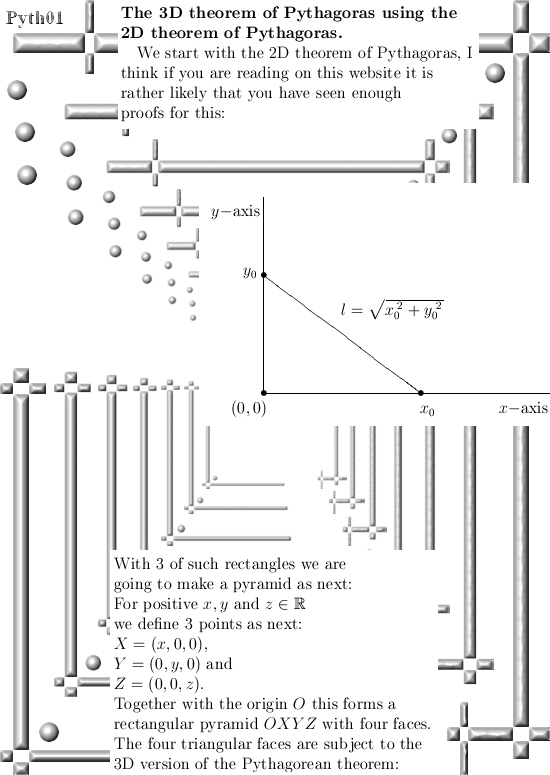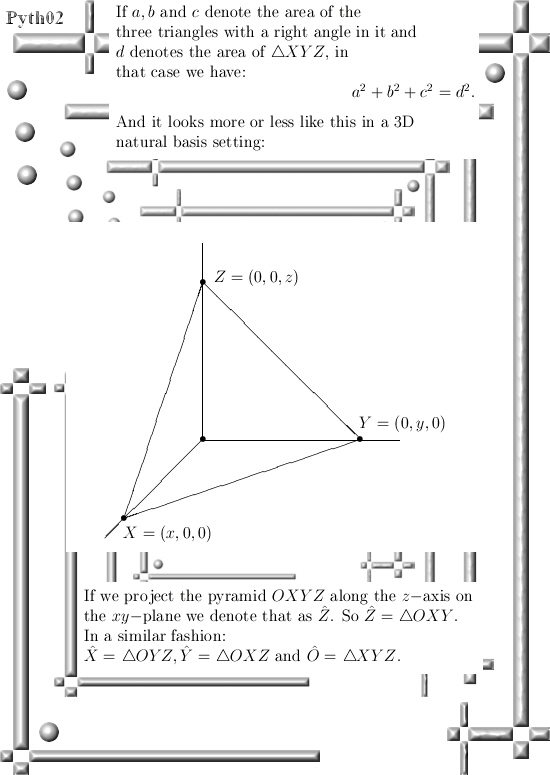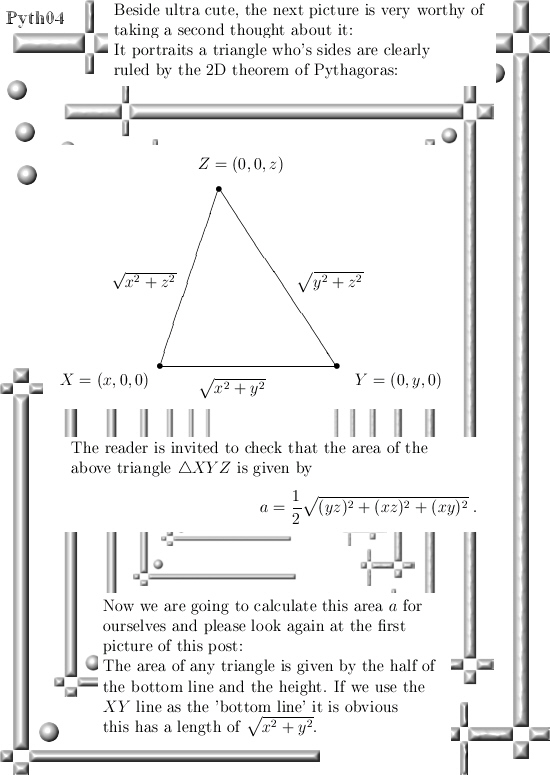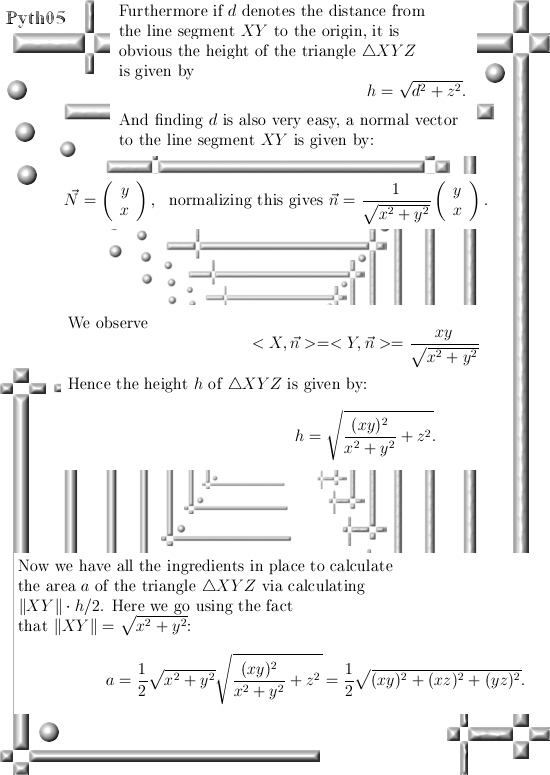# General Pythagoras theorem part 1: The 3D case.

A long time ago I found a very simple proof for the general theorem Pythagoras. At the time the general public had almost zero access to internet resources and in those long lost years I could not find out if my proof was found yes or no.

As memory serves, Descartes was the one that gave a proof for the 3D version of the Pythagorean theorem… (But I never did read the proof of Descartes.)

Two weeks back I was cleaning out my book closet so I could store more bottles of beer for the ripening process and I came across that old but never perfectly finished proof.

And it entered my mind again because it is fascinating that just by constructing that perfect normal vector, you make it of unit length, calculate a few higher dimensional volumes and voila:
There is you proof of the general theorem of Pythagoras.

In this post we only look at the 3D example for the theorem of Pythagoras. But already here we use a normal vector together with the 2D theorem of Pythagoras in order to prove the result for 3D space.
Basically this is also precisely the way the proof works in all higher dimensions, ok ok the notations and ways of writing the stuff down is a bit more technical but if you understand the proof in this post you will immediately understand how the general proof works.

The general proof is based on the principle of natural induction, likely the reader is familiar with natural or mathematical induction because beside it’s elegance it is also easy to explain to first year students in exact sciences. Basically you prove some stuff for low values of n, say n = 2 or 3 for 2D and 3D space and after that you do the so called ‘induction step’ where you must show that if it holds for a particular value of n, the stuff you want to prove is also true for n + 1.

Here is a wiki on the subject: Mathematical induction
https://en.wikipedia.org/wiki/Mathematical_induction

__________

This post is five pictures long (size 550 x 775 as usual) so have fun reading it:In the last line of the proof it is important to remark that both the length of XY is done with the 2D version of Pythagoras, but the height h of triangle XYZ is also done with the 2D version of Pythagoras. And so you get the 3D version of the famous Pythagoras theorem.

See you in the next post where it is all a bit more abstract and not slammed down to just two or three dimensions. Have a nice life or try to get one.